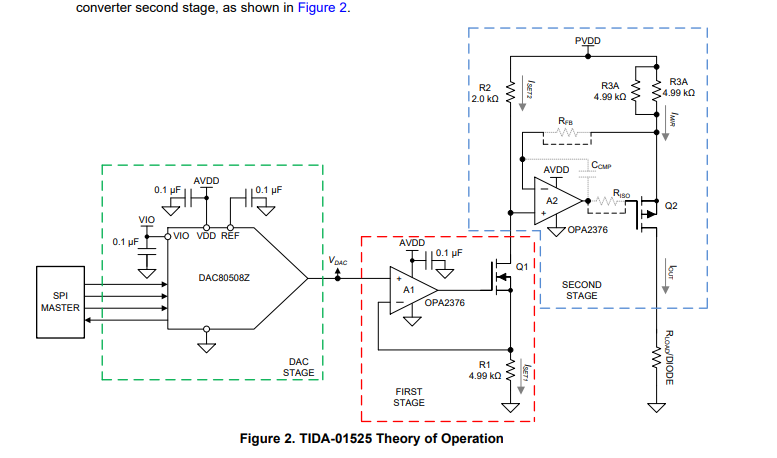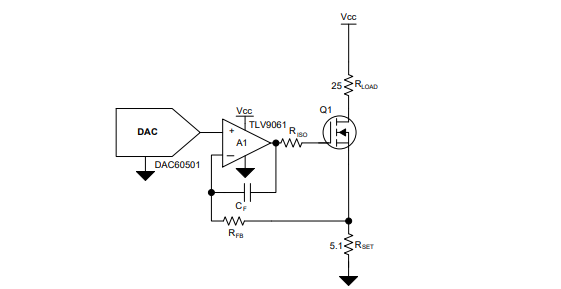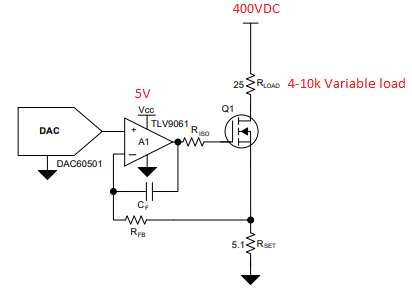If you have a related question, please click the "Ask a related question" button in the top right corner. The newly created question will be automatically linked to this question.

• Resolved

# TIDA-01525: TIDA-01525

Part Number: TIDA-01525

Hello,

Can I set the voltage to 400 Volts for this circuit. Of course, I will use the flyback converter for 400 volt DC. I need: 0-200mA adjustable current source for 400 Volts.

• Hi Alpay,

This change cannot be easily made. Specifically, this is a "high-side current source", meaning that that PVDD would need to be 400V, and that the op amp regulating the current would need to be biased at that high voltage.

A better solution would be to implement a low side current source, which is basically just the first segment of the circuit (the green and red regions).There is a good cookbook design showing this: http://www.ti.com/lit/an/slaa868/slaa868.pdf

They are using the single-channel version, but an 8-channel version is easily created from the same design.In this case, the TLV9061 (or any unipolar amp with low offset voltage) would be powered from some low voltage supply (~5V), and the high side voltage (connected to Rload) would be 400V.

Hope this helps!

Thanks,

Paul

Hello paul,

The solution you're suggesting looks great.

To be sure, I want to ask; Can I give this circuit 400V from Vcc line? It's great if it's such a simple solution!

Thanks,

Alpay,

• In reply to Alpay Zeren:

The amplifier's supply cannot be 400V, but the load's high side supply can be.  You will also need to adjust the resistance values to ensure that your current range is good.  You will also need to find a FET (Q1) that is rated for that voltage.  Otherwise, the schematic will be the same.

Thanks,

Paul

Hello Paul,
I have one last question; The load variable I use will be 4K to 10K. In this case, I expect the supply voltage to change and the current to be constant. Is this true ?

Thank you very much for your interest.• In reply to Alpay Zeren:

If your load changes and the 400V supply is static, the circuit would maintain constant current (200mA, for example).  The total power in the circuit would be constant (Power_total = V×I = 400V × 200mA = 80 W).  When you Rload value changes, you will see this power in the different segments change.  For example, if your load was 1Ω, then Pload = 200mW.  The other 79.8W would be dissipated in Q1 and Rset.  For this reason, you will need Q1 to be capable of handling this power.

Ideally, you would scale the PVDD supply (400V) to the minimum value you need to have the desired power across the load.